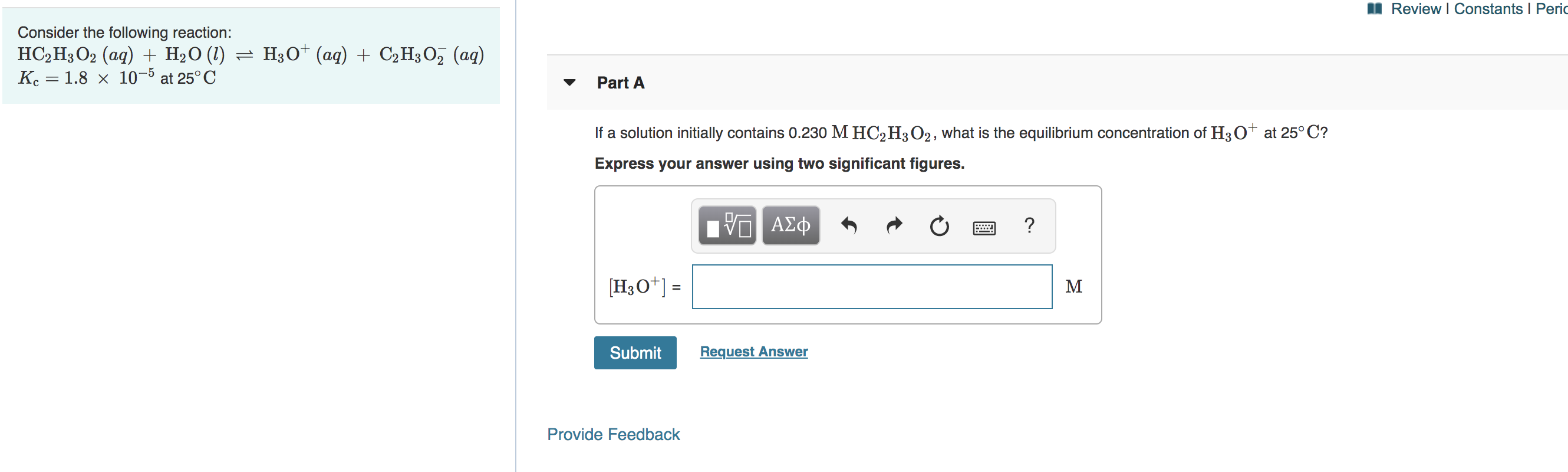# Consider the following reaction: HC2H3O2(aq) + H2O(l) ⇌ H3O+(aq) + C2H3O2-(aq) Kc = 1.8×10-5 at 25°C. If a solution initially contains 0.230 M HC2H3O2, what is the equilibrium concentration of H3O^+ at 25°C? Express your answer using two significant figures.